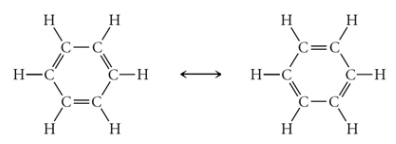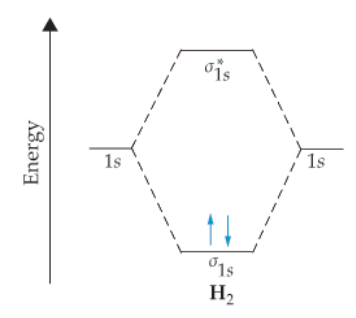# Problem: In the benzene molecule, carbon atoms form a ring with alternating single and double bonds in between each of them. Each carbon atom forms one σ bond with one carbon atom and one σand one π bond with another. The π bonding electrons are delocalized over the entire molecule. Thus, benzene is a resonance hybrid of two equivalent structures, and the single and double bonds oscillate from one position to the other.Calculate the bond order for one carbon-carbon bond in the benzene molecule, taking σ and π bonding into consideration.Express your answer numerically.Chemical bonds are formed by linear combinations of atomic orbitals. These atomic orbitals should have compatible energies. An axial overlap of these orbitals results in the formation of a σ bond, whereas a lateral overlap results in the formation of a π bond.When two atomic orbitals on different atoms approach each other, two molecular orbitals are formed: one bonding molecular orbital and one antibonding molecular orbital. The bonding molecular orbital has a lower energy than the corresponding atomic orbitals; antibonding orbitals have higher energies than the corresponding atomic orbitals. An example using the hydrogen molecule is shown here. However, the same rule applies to systems with multiple atomic orbitals. For each atomic orbital that overlaps, one molecular orbital must form. In the diagram below, 1s orbitals from two hydrogen atoms combine to form one bonding σ1s orbital and one antibonding σ1s* orbital. The total number of bonding and antibonding orbitals is always equal to the number of orbitals that overlap.Similarly, molecular orbitals can also be formed by the overlapping of hybrid orbitals. The hybrid orbitals are formed by the linear combination of different atomic orbitals of nearly equal energy on the same atom. The atomic orbitals of nearly equal energy undergo a process of mixing and reorientation to form new orbitals of lower energy. This process is called hybridization. For example, hybridization of one s and two p orbitals on an atom forms three sp2 hybrid orbitals.

###### FREE Expert Solution
98% (145 ratings)View Complete Written Solution
###### Problem Details

In the benzene molecule, carbon atoms form a ring with alternating single and double bonds in between each of them. Each carbon atom forms one σ bond with one carbon atom and one σand one π bond with another. The π bonding electrons are delocalized over the entire molecule. Thus, benzene is a resonance hybrid of two equivalent structures, and the single and double bonds oscillate from one position to the other.Calculate the bond order for one carbon-carbon bond in the benzene molecule, taking σ and π bonding into consideration.

Chemical bonds are formed by linear combinations of atomic orbitals. These atomic orbitals should have compatible energies. An axial overlap of these orbitals results in the formation of a σ bond, whereas a lateral overlap results in the formation of a π bond.

When two atomic orbitals on different atoms approach each other, two molecular orbitals are formed: one bonding molecular orbital and one antibonding molecular orbital. The bonding molecular orbital has a lower energy than the corresponding atomic orbitals; antibonding orbitals have higher energies than the corresponding atomic orbitals. An example using the hydrogen molecule is shown here. However, the same rule applies to systems with multiple atomic orbitals. For each atomic orbital that overlaps, one molecular orbital must form. In the diagram below, 1s orbitals from two hydrogen atoms combine to form one bonding σ1s orbital and one antibonding σ1s* orbital. The total number of bonding and antibonding orbitals is always equal to the number of orbitals that overlap.Similarly, molecular orbitals can also be formed by the overlapping of hybrid orbitals. The hybrid orbitals are formed by the linear combination of different atomic orbitals of nearly equal energy on the same atom. The atomic orbitals of nearly equal energy undergo a process of mixing and reorientation to form new orbitals of lower energy. This process is called hybridization. For example, hybridization of one s and two p orbitals on an atom forms three sp2 hybrid orbitals.